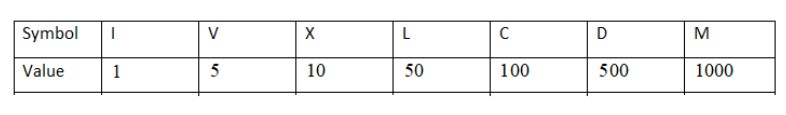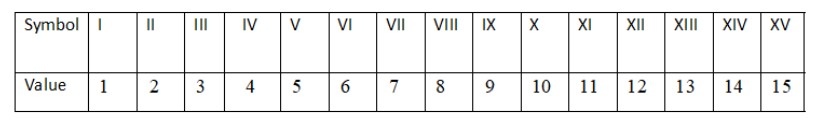Write the numbers using Roman numerals.1) $9$2) $2$3) $4$4) $11$Verified
147k+ views
Hint:In this question we have to write the given numbers in roman numerals.Numbers in this system are represented by combinations of letters from the Latin alphabet. Roman numerals, as used today, are based on seven symbols:Formula used:
The numbers $1$ to $10$ are usually expressed in Roman numerals as follows: ${\mathbf{I}},{\text{ }}{\mathbf{II}},{\text{ }}{\mathbf{III}},{\text{ }}{\mathbf{IV}},{\text{ }}{\mathbf{V}},{\text{ }}{\mathbf{VI}},{\text{ }}{\mathbf{VII}},{\text{ }}{\mathbf{VIII}},{\text{ }}{\mathbf{IX}},{\text{ }}{\mathbf{X}}$.

We need to write the numbers $9,2,4,11$ using Roman numerals.
The number $9$ is written $IX$ using Roman Numerals
The number $2$ is written $II$ using Roman Numerals
The number$4$ is written $IV$ using Roman Numerals
The number $11$ is written as $10 + 1$ so $XI$ using Roman Numerals (As $10$ is written as $X\,\&\, 1$ is written as $I$)
Thus, the numbers are written using Roman numerals as
$(1)\,9 ={IX}$
$(2)\,2 ={II}$
$(4 )\,4 ={IV}$
$(5)\,11 ={XI}$

Additional Information:Roman numerals are used in astronomy to designate moons and in chemistry to denote groups of the periodic table. They can be seen in tables of contents and in manuscript outlines, as upper- and lower-case Roman numerals break information into an easily organized structure. Music theory employs Roman numerals in notation symbols.

Note:The use of Roman numerals continued long after the decline of the Roman Empire. From the ${14^{th}}$ century on, Roman numerals began to be replaced in most contexts by the more convenient Hindu-Arabic numerals; however, this process was gradual, and the use of Roman numerals persists in some minor applications to this day. The numbers $1$ to $15$ are usually expressed in Roman numerals as follows: